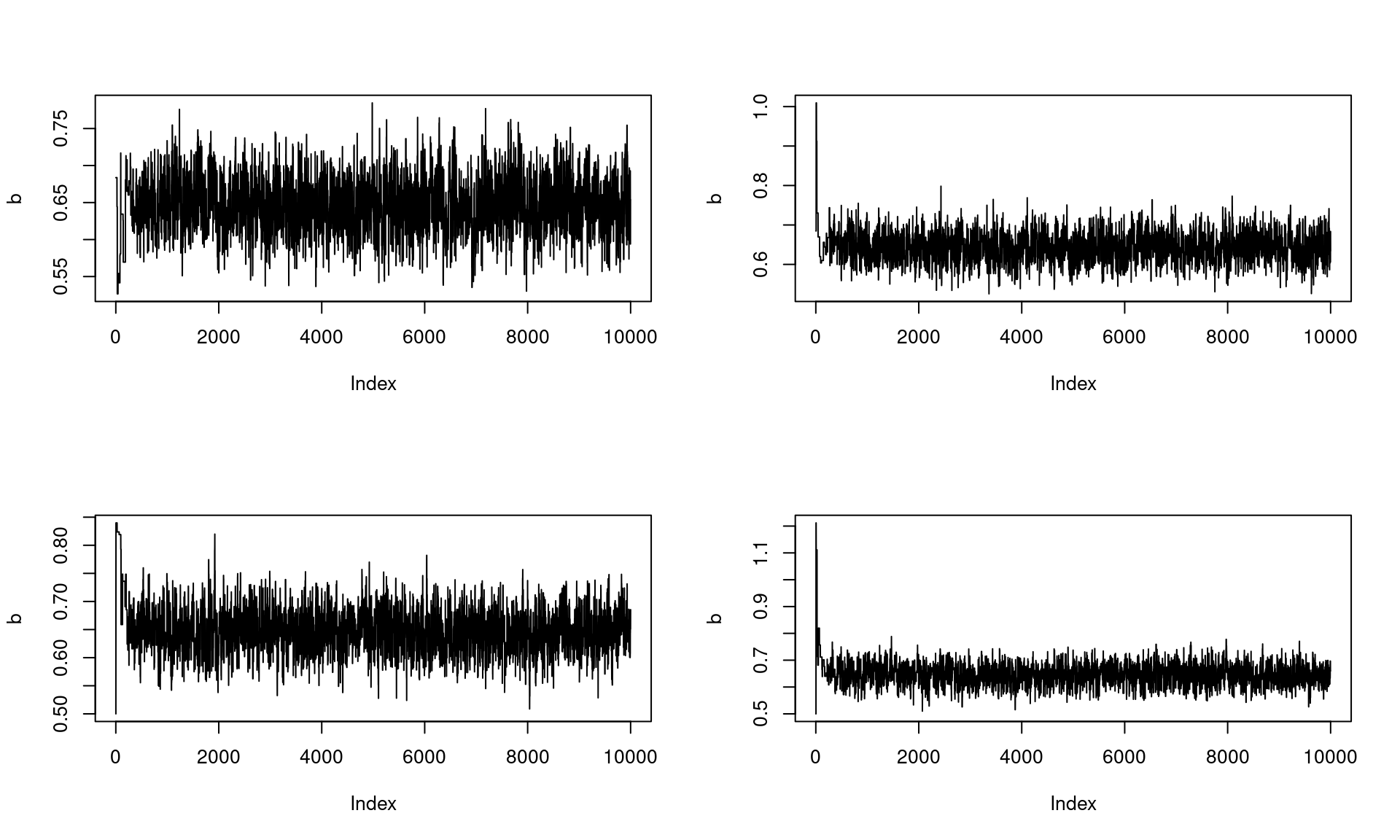### Overview

Parallelization is a useful feature of multi-core processors that improves computation time by running multiple independent processes simultaneously.

Due to how NIMBLE builds models and algorithms and automatically generates C++ code, parallelizating NIMBLE code requires some care. Naive approaches such as building a single model using standard parallelization packages in R to parallelize an MCMC are likely to fail, or worse, behave incorrectly without failing.

That said, parallelizing NIMBLE pipelines is possible and can be very convenient if done properly. The key consideration is to ensure that all NIMBLE execution, including model building, is conducted inside the parallelized code. This ensures that all models and algorithms are independent objects that don’t interfere with each other.

Note that we have work in progress to make parallelizing NIMBLE code easier and more powerful.

### Parallelization in practice

In this example, we’ll use basic functionality from the parallel package to run MCMC in parallel chains.

library(parallel)

First we create a cluster, specifying the number of cores we want the cluster to operate across.

this_cluster <- makeCluster(4)

(Side note: do not use makeForkCluster when parallelizing NIMBLE code; it will not work. makeCluster sets up a PSOCK cluster, which will work. If you really want to use forking, if you are able to set up separate directories for NIMBLE’s generated code for each worker, that should allow one to use forked processes. One option is to use unixtools::set.tempdir() and another is to use the dirName argument to compileNimble().)

We’ll set up a situation where we run four independent MCMC simulations.

The key is to create a function that includes all the modeling steps and run that function in parallel. Note that myCode could have been created outside the parallelized code, but all the other steps need to be done inside the parallelized code.

set.seed(10120)
# Simulate some data
myData <- rgamma(1000, shape = 0.4, rate = 0.8)

# Create a function with all the needed code
run_MCMC_allcode <- function(seed, data) {
library(nimble)

myCode <- nimbleCode({
a ~ dunif(0, 100)
b ~ dnorm(0, 100)

for (i in 1:length_y) {
y[i] ~ dgamma(shape = a, rate = b)
}
})

myModel <- nimbleModel(code = myCode,
data = list(y = data),
constants = list(length_y = 1000),
inits = list(a = 0.5, b = 0.5))

CmyModel <- compileNimble(myModel)

myMCMC <- buildMCMC(CmyModel)
CmyMCMC <- compileNimble(myMCMC)

results <- runMCMC(CmyMCMC, niter = 10000, setSeed = seed)

return(results)
}

Now, we execute the desired code using parLapply, which is equivalent to lapply with each process running in parallel.

Alternatively, we could use foreach with the doParallel backend (via registerDoParallel, which uses makeCluster by default under the hood) to invoke run_MCMC_allcode in parallel.

chain_output <- parLapply(cl = this_cluster, X = 1:4,
fun = run_MCMC_allcode,
data = myData)

# It's good practice to close the cluster when you're done with it.
stopCluster(this_cluster)

We ran four independent MCMCs.

par(mfrow = c(2,2))
for (i in 1:4) {
this_output <- chain_output[[i]]
plot(this_output[,"b"], type = "l", ylab = 'b')
}### Using user-defined distributions, functions, or samplers with parallelization

Note that NIMBLE generally expects user-defined distributions, functions, and samplers to be defined in the global environment. As discussed above, for parallelization, it is important to do all the model and MCMC building and compilation inside a function. Therefore, in order to achieve this but still have the user-defined elements in the global environment we recommend using assign to copy them into the global environment from within your function.

After defining your distribution, function, or sampler inside your overall function, here is how you can do that:

## for a user-defined function
assign('dfoo', dfoo, envir = .GlobalEnv)
assign('rfoo', rfoo, envir = .GlobalEnv)
## for a user-defined distribution
assign('myfun', myfun, envir = .GlobalEnv)
## for a user-defined sampler
assign('sampler_mySampler', sampler_mySampler, envir = .GlobalEnv)